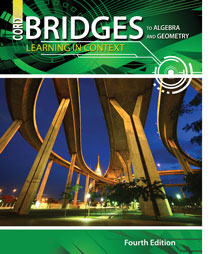# Bridges to Algebra and Geometry - 4th Edition

## Chapter 4: Solving Equations

Some links are repeated for use with more than one lesson.

### The following links apply to the entire chapter.

http://www.algebrahelp.com/lessons/equationbasics/index.htm
This site defines the parts of an equation and shows how to solve them.

http://www.explorelearning.com/index.cfm?method=cResource.dspResourcesForCourse&CourseID=216
This site has examples and problems for students to solve that involve using expressions, solving equations, and inequalities.

http://www.sosmath.com/algebra/solve/solve0/solve0.html#linear
This site has problems for the students to solve that involve linear equations, absolute values, quadratic equations, and more.

### 4.1 Addition and Subtraction Equations

http://www.algebrahelp.com/lessons/equationbasics/pgw.htm
This site has a worksheet for students to solve that involves basic equations..

### 4.2 Multiplication and Division Equations

http://www.algebrahelp.com/lessons/equationbasics/pgw.htm
This site has a worksheet for students to solve that involves basic equations.

http://www.mathwords.com/e/equation_rules.htm
This site summarizes the properties of equality that we use to solve equations.

### 4.3 Two-Step Equations

NEW This site presents the multiplication and division properties combined with the addition and subtraction properties of equality to solve equations.

### 4.4 Adding and Subtracting Expressions

http://www.freemathhelp.com/combining-like-terms.html
NEW This site shows how like terms can be combined to simplify an equation.

### 4.5 Equations with Variables on Both Sides

http://www.algebrahelp.com/worksheets/view/simplifying/combiningliketerms.quiz
NEW This site provides practice problems for combining like terms from both sides of the equation, to solve for the unknown variable.

### 4.6 Formulas

http://www.purplemath.com/modules/solvelin.htm
NEW This four-page series reviews the steps to solve almost any equation involving addition/subtraction and multiplication/division..